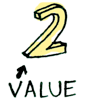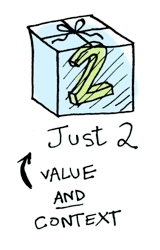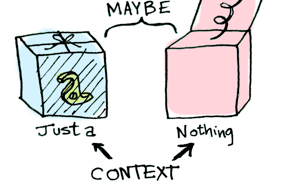sealed class Maybe<out T> {
object `Nothing#` : Maybe<Nothing>() {
override fun toString(): String = "Nothing#"
}
data class Just<out T>(val value: T) : Maybe<T>()
}

Functor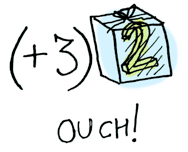> Maybe.Just(2).fmap { it + 3 }
Just(value=5)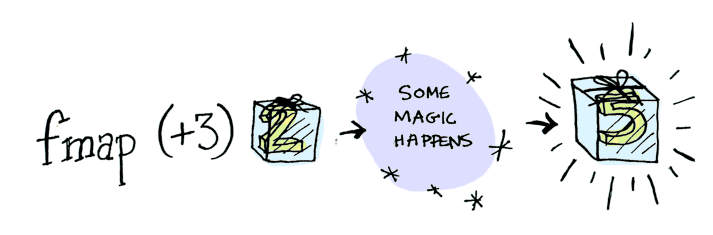究竟什么是 Functor 呢？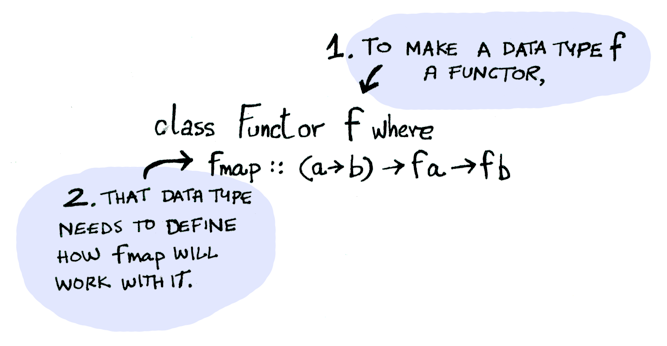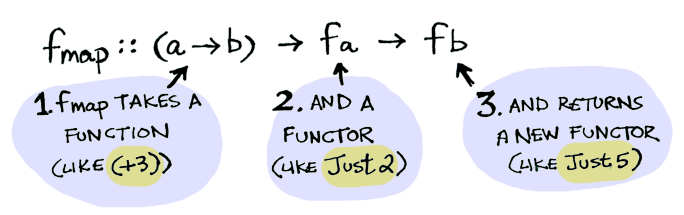> Maybe.Just(2).fmap { it + 3 }
Just(value=5)

fmap 神奇地应用了这个函数，因为 Maybe 是一个 Functor。 它指定了 fmap 如何应用到 Just 上与 Nothing# 上：

fun <T, R> Maybe<T>.fmap(transform: (T) -> R): Maybe<R> = when(this) {
Maybe.`Nothing#` -> Maybe.`Nothing#`
is Maybe.Just -> Maybe.Just(transform(this.value))
}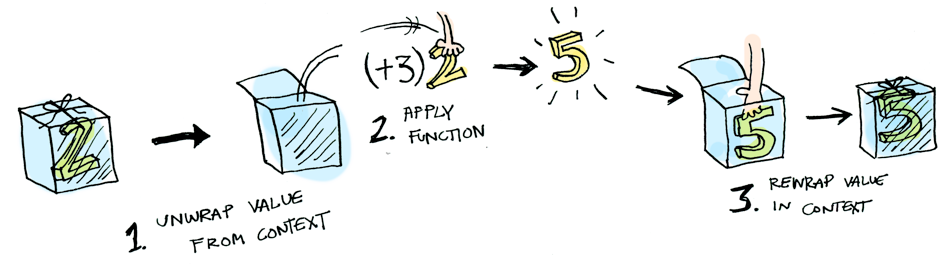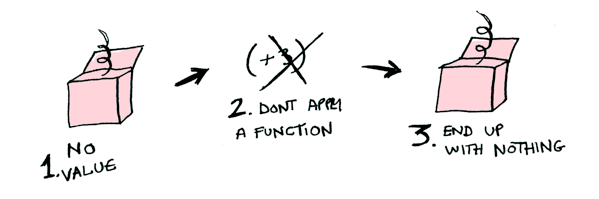> Maybe.`Nothing#`.fmap { x: Int -> x + 3 }
Nothing#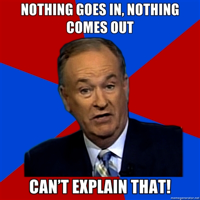post = Post.find_by_id(1)
if post
return post.title
else
return nil
end

findPost(1).fmap(::getPostTitle)

infix fun <T, R> ((T) -> R).`(\$)`(maybe: Maybe<T>) = maybe.fmap(this)

::getPostTitle `(\$)` findPost(1)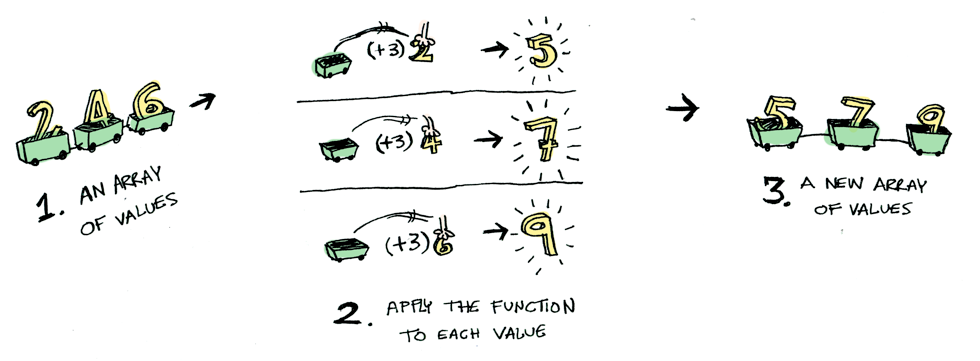Iterable 也是 functor！ 我们可以为其定义 fmap 如下：

fun <T, R> Iterable<T>.fmap(transform: (T) -> R): List<R> = this.map(transform)

{ x: Int - > x + 1 }.fmap { x: Int -> x + 3 }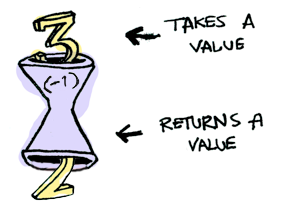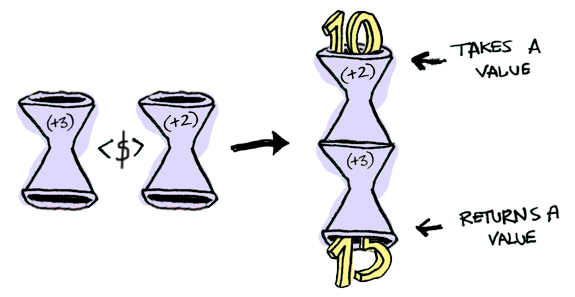> fun <T, U, R> ((T) -> U).fmap(transform: (U) -> R) = { t: T -> transform(this(t)) }
> val foo = { x: Int -> x + 2 }.fmap { x: Int -> x + 3 }
> foo(10)
15

Applicative

Applicative 又提升了一个层次。 对于 Applicative，我们的值像 Functor 一样包装在一个上下文中：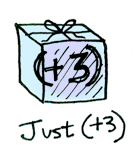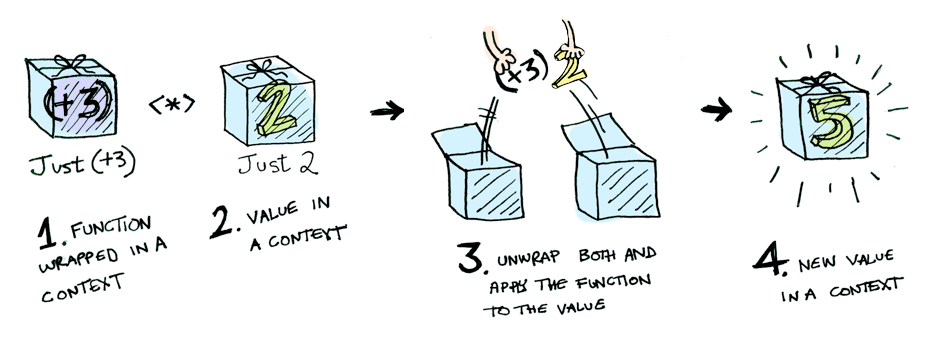infix fun <T, R>  Maybe<(T) -> R>.`(*)`(maybe: Maybe<T>): Maybe<R> = when(this) {
Maybe.`Nothing#` -> Maybe.`Nothing#`
is Maybe.Just -> this.value `(\$)` maybe
}

Maybe.Just { x: Int -> x + 3 } `(*)` Maybe.Just(2) == Maybe.Just(5)

infix fun <T, R>  Iterable<(T) -> R>.`(*)`(iterable: Iterable<T>) = this.flatMap { iterable.map(it) }

> listOf<(Int) -> Int>({ it * 2 }, { it + 3 }) `(*)` listOf(1, 2, 3)
[2, 4, 6, 4, 5, 6]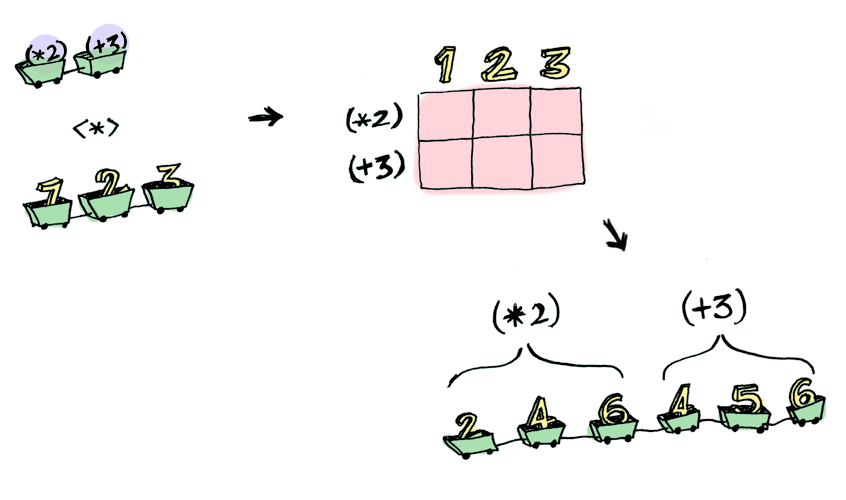> { y: Int -> { x: Int -> x + y } } `(\$)` Maybe.Just(5)
Just(value=(kotlin.Int) -> kotlin.Int) // 等于 `Maybe.Just { x: Int -> x + 5 }`
> Maybe.Just { x: Int -> x + 5 } `(\$)` Maybe.Just(4)

Applicative：

> { y: Int -> { x: Int -> x + y } } `(\$)` Maybe.Just(5)
Just(value=(kotlin.Int) -> kotlin.Int) // 等于 `Maybe.Just { x: Int -> x + 5 }`
> Maybe.Just { x: Int -> x + 5 } `(*)` Maybe.Just(3)
Just(value=8)

ApplicativeFunctor 推到一边。 “大人物可以使用具有任意数量参数的函数，”它说。 “装备了 (\$)(*) 之后，我可以接受具有任意个数未包装值参数的任意函数。 然后我传给它所有已包装的值，而我会得到一个已包装的值出来！ 啊啊啊啊啊！”

> { y: Int -> { x: Int -> x + y } } `(\$)` Maybe.Just(5) `(*)` Maybe.Just(3)
Just(value=15)

fun <T> ((x: T, y: T) -> T).liftA2(m1: Maybe<T>, m2: Maybe<T>) =
{ y: T -> { x: T -> this(x, y) } } `(\$)` m1 `(*)` m2

> { x: Int, y: Int -> x * y }.liftA2(Maybe.Just(5), Maybe.Just(3))
Just(value=15)

1. 取得计算机科学博士学位。
2. 然后把它扔掉，因为在本节中你并不需要！

Functor 将一个函数应用到一个已包装的值上：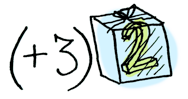Applicative 将一个已包装的函数应用到一个已包装的值上：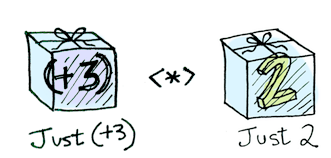fun half(x: Int) = if (x % 2 == 0)
Maybe.Just(x / 2)
else
Maybe.`Nothing#`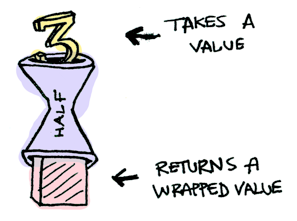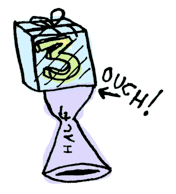> Maybe.Just(3) `))=` ::half
Nothing#
> Maybe.Just(4) `))=` ::half
Just(value=2)
> Maybe.`Nothing#` `))=` ::half
Nothing#

(>>=) :: m a -> (a -> m b) -> m b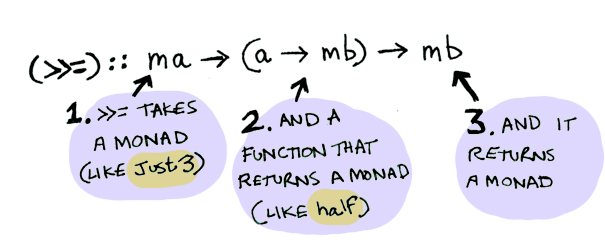infix fun <T, R> Maybe<T>.`))=`(f: ((T) -> Maybe<R>)): Maybe<R> = when(this) {
Maybe.`Nothing#` -> Maybe.`Nothing#`
is Maybe.Just -> f(this.value)
}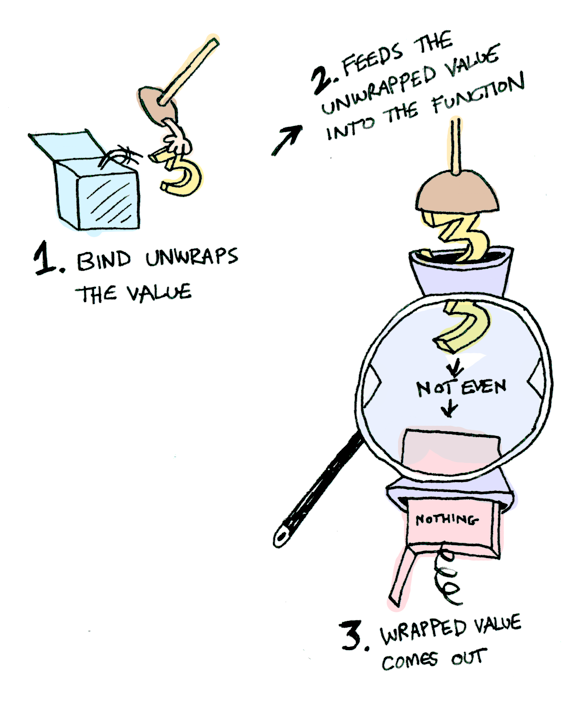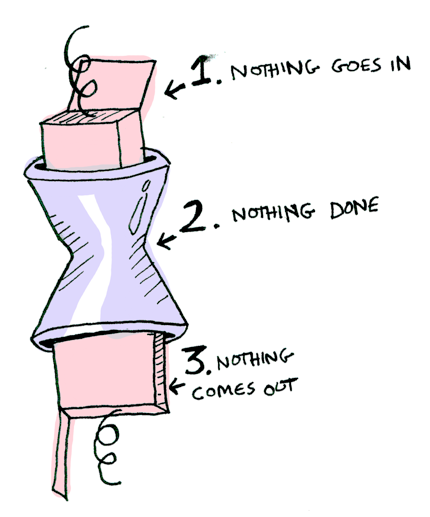> Maybe.Just(20) `))=` ::half `))=` ::half `))=` ::half
Nothing#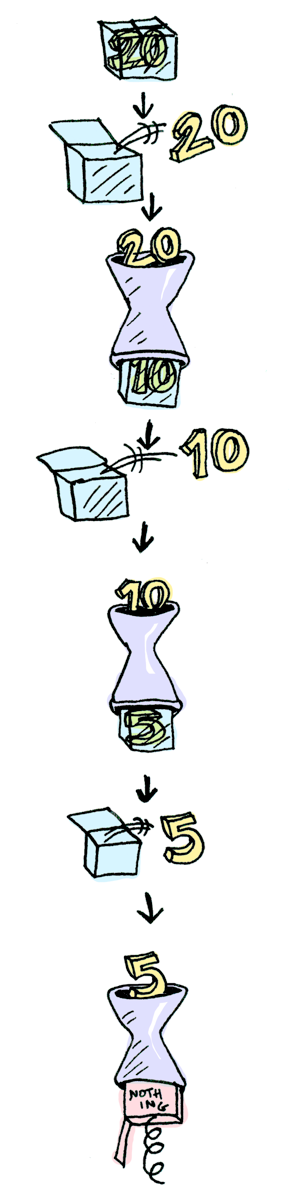fun Int.half() = if (this % 2 == 0) this / 2 else null

val n: Int? = 20
n?.half()?.half()?.half()data class IO<out T>(val `(-`: T)

infix fun <T, R> IO<T>.`))=`(f: ((T) -> IO<R>)): IO<R> = f(this.`(-`)fun getLine(): IO<String> = IO(readLine() ?: "")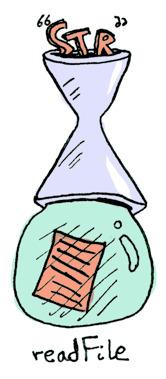typealias FilePath = String

putStrLn 接受一个字符串并输出之：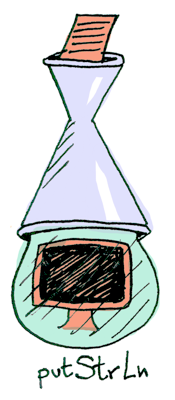fun putStrLn(str: String): IO<Unit> = IO(println(str))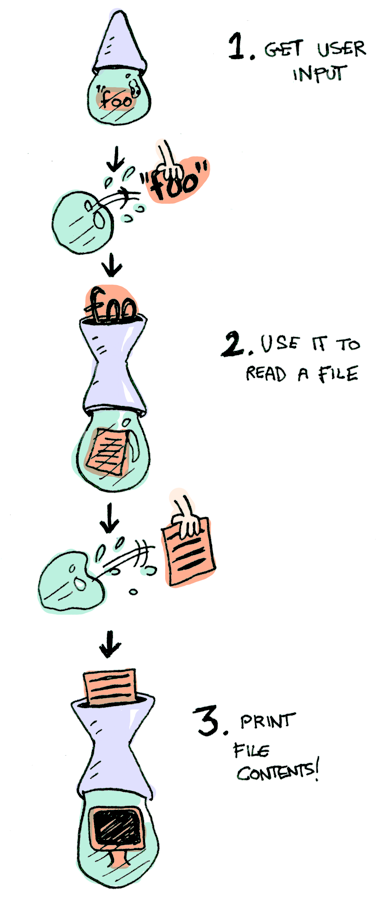foo = do
filename <- getLine
putStrLn contents

fun <T> `do` (ioOperations: () -> IO<T>) = ioOperations()

val foo = `do` {
val filename = getLine().`(-`
putStrLn(contents)
}

结论

1. （Haskell 中的）functor 是实现了 Functor 类型类的数据类型。
2. （Haskell 中的）applicative 是实现了 Applicative 类型类的数据类型。
4. Maybe 实现了这三者，所以它是 functor、 applicative、 以及 monad。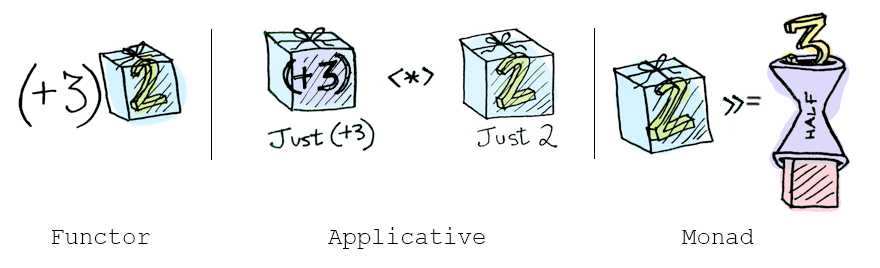• functor: 可通过 fmap 或者 (\$) 将一个函数应用到一个已包装的值上。
• applicative: 可通过 (*) 或者 liftA 将一个已包装的函数应用到已包装的值上。
• monad: 可通过 ))= 或者 liftM 将一个返回已包装值的函数应用到已包装的值上。